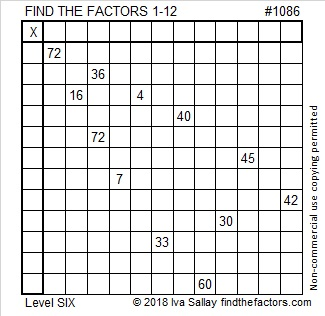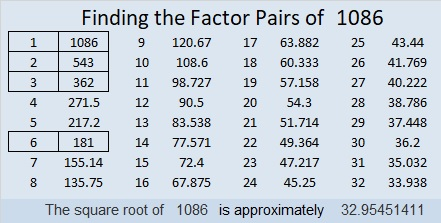# 1086 and Level 6

The Find the Factors 1-12 puzzles allow 36 and 72 to use three common factors: 6, 9, and 12. Which one should you choose?  Likewise, 16 and 4’s permitted common factors are 2 and 4.

Sure you could guess and check to solve this puzzle, but that might frustrate you. I assure you that you can solve this puzzle by using logic.

If you get stuck getting started, here are a couple of things to consider:
Can both 72’s in the puzzle be 8×9? How about 6×12?
(36 = 3 × 12), (30 = 3 × 10), and (33 = 3 × 11) Which two of those clues MUST use both of the 3’s?Print the puzzles or type the solution in this excel file: 12 factors 1080-1086

Now I’ll share a few facts about the number 1086:

• 1086 is a composite number.
• Prime factorization: 1086 = 2 × 3 × 181
• The exponents in the prime factorization are 1, 1, and 1. Adding one to each and multiplying we get (1 + 1)(1 + 1)(1 + 1) = 2 × 2 × 2 = 8. Therefore 1086 has exactly 8 factors.
• Factors of 1086: 1, 2, 3, 6, 181, 362, 543, 1086
• Factor pairs: 1086 = 1 × 1086, 2 × 543, 3 × 362, or 6 × 181
• 1086 has no square factors that allow its square root to be simplified. √1086 ≈ 32.954511086 is the hypotenuse of a Pythagorean triple:
114-1080-1086 which is 6 times (19-180-181)

1086 is a palindrome in three other bases:
It’s 8A8 in BASE 11 (A is 10 base 10) because 8(121) + 10(11) + 8(1) = 1086,
303 in BASE 19 because 3(19² + 1) = 1086, and
141 in BASE 31 because 1(31²) + 4(31) + 1(1) = 1086

This site uses Akismet to reduce spam. Learn how your comment data is processed.